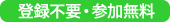### 第5回 統計的機械学習セミナー／5th Statistical Machine Learning Seminar （主催：統計数理研究所 統計的機械学習NOE)

http://noe.ism.ac.jp/sml/seminar/2011-09-02-seminar/

2011年9月2日（金） 15:00-17:0015:00-16:00
Guido Montúfar
(Max Planck Institute for Mathematics in the Sciences)
Title
Geometry and Approximation Errors of Restricted Boltzmann Machines
Abstract
Restricted Boltzmann machines are used as training blocks for deep belief nets, which on the other hand have shown to be promising models for capturing the complicated structure of high-dimensional real world data. In reverse, the geometry of these models is complicated. In this talk I discuss the geometry of restricted Boltzmann machines and features that they can capture in such a way as to assess approximation errors and to provide a basis for risk minimization in this class of models.
16:00-17:00
Jun Zhang (Department of Psychology, University of Michigan)
Title
Regularized Learning in Reproducing Kernel Banach Spaces
Abstract
Regularized learning is the contemporary framework for learning to generalize from finite samples (classification, regression, clustering, etc). Here the problem is to learn an input-output mapping f: X->Y, either scalar-valued or vector-valued, given finite samples {(xi, yi), i=1,…,N}. With minimal structural assumptions on X, the class of functions under consideration is assumed to fall under a Banach (especially, Hilbert) space of functions B. The learning-from-data problem is then formulated as an optimization problem in such a function space, with the desired mapping as an optimizer to be sought, where the objective function consists of a loss term L(f) capturing its goodness-of-fit (or the lack thereof) on given samples {(f(xi), yi), i=1,…,N}, and a penalty term R(f) capturing its complexity based on prior knowledge about the solution (smoothness, sparsity, etc). This second, regularizing term is often taken to be the norm of B, or an innocent transformation Φ thereof: R(f) = Φ(||f||). This program has been successfully carried out for the Hilbert space of functions, resulting in the celebrated Reproducing Kernel Hilbert Space methods in machine learning. Here, we will remove the Hilbert space restriction, i.e., the existence of an inner product, and show that the key ingredients of this framework (reproducing kernel, representer theorem, feature space) remain to hold for a Banach space that is uniformly convex and uniformly Frechet differentiable. Central to our development is the use of a semi-inner product operator and duality mapping for a uniform Banach space in place of an inner-product for a Hilbert space. This opens up the possibility of unifying kernel-based methods (regularizing L2-norm) and sparsity-based methods (regularizing l1-norm), which have so far been investigated under different theoretical foundations.
▲ このページのトップへ